# The interest rate on a \$64,000 loan is 9.6% compounded semiannually. Quarterly payments will pay off...

 The interest rate on a \$64,000 loan is 9.6% compounded semiannually. Quarterly payments will pay off the loan in fifteen years. (Do not round intermediate calculations and round your final answers to 2 decimal places.)
 a. Calculate the interest component of Payment 13.
 Interest \$

 b. Calculate the principal component of Payment 52.
 Principal \$

 c. Calculate the total interest in Payments 41 to 50 inclusive.
 Total interest \$

 d. Calculate the reduction of principal in Year 5.
 Principal reduction \$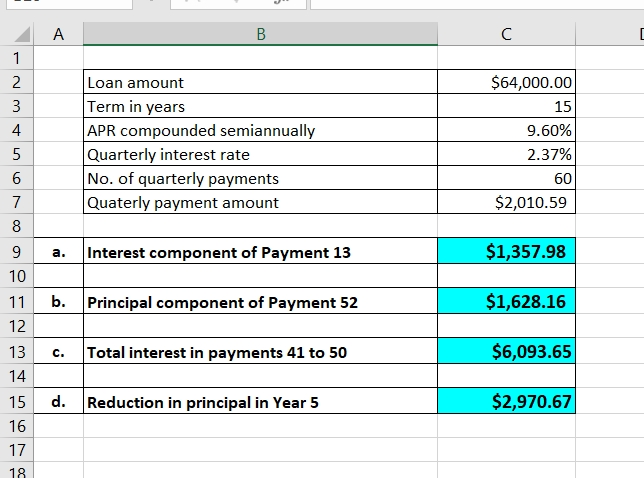Cell reference -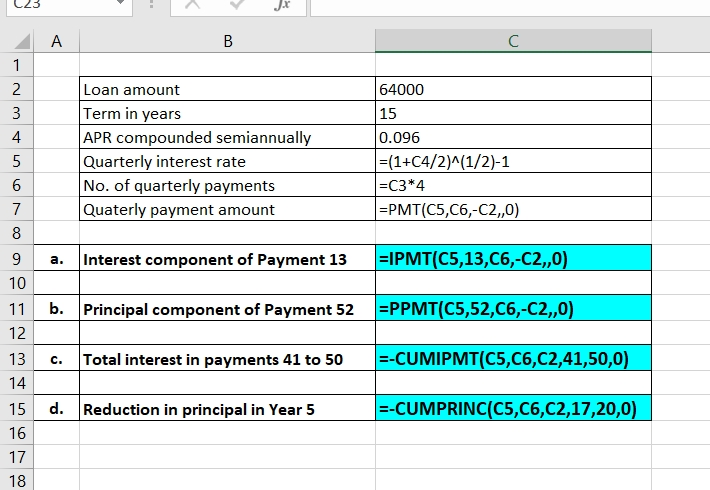Hope it will help, please do comment if you need any further explanation. Your feedback would be highly appreciated.

##### Add Answer of: The interest rate on a \$64,000 loan is 9.6% compounded semiannually. Quarterly payments will pay off...
Similar Homework Help Questions
• ### The interest rate on a \$60,000 loan is 9.1% compounded semiannually. Quarterly payments will pay off...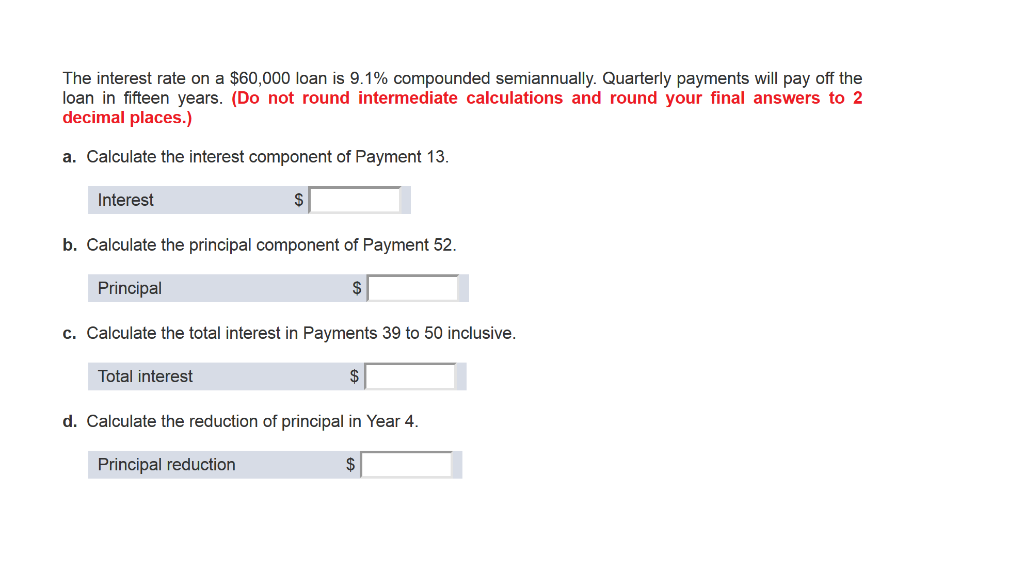The interest rate on a \$60,000 loan is 9.1% compounded semiannually. Quarterly payments will pay off the loan in fifteen years. (Do not round intermediate calculations and round your final answers to 2 decimal places.) a. Calculate the interest component of Payment 13. Interest \$ b. Calculate the principal component of Payment 52. Principal \$ c. Calculate the total interest in Payments 39 to 50 inclusive. Total interest d. Calculate the reduction of principal in Year 4. Principal reduction \$

• ### A \$27,000 loan at 12% compounded quarterly is to be repaid by equal quarterly payments over...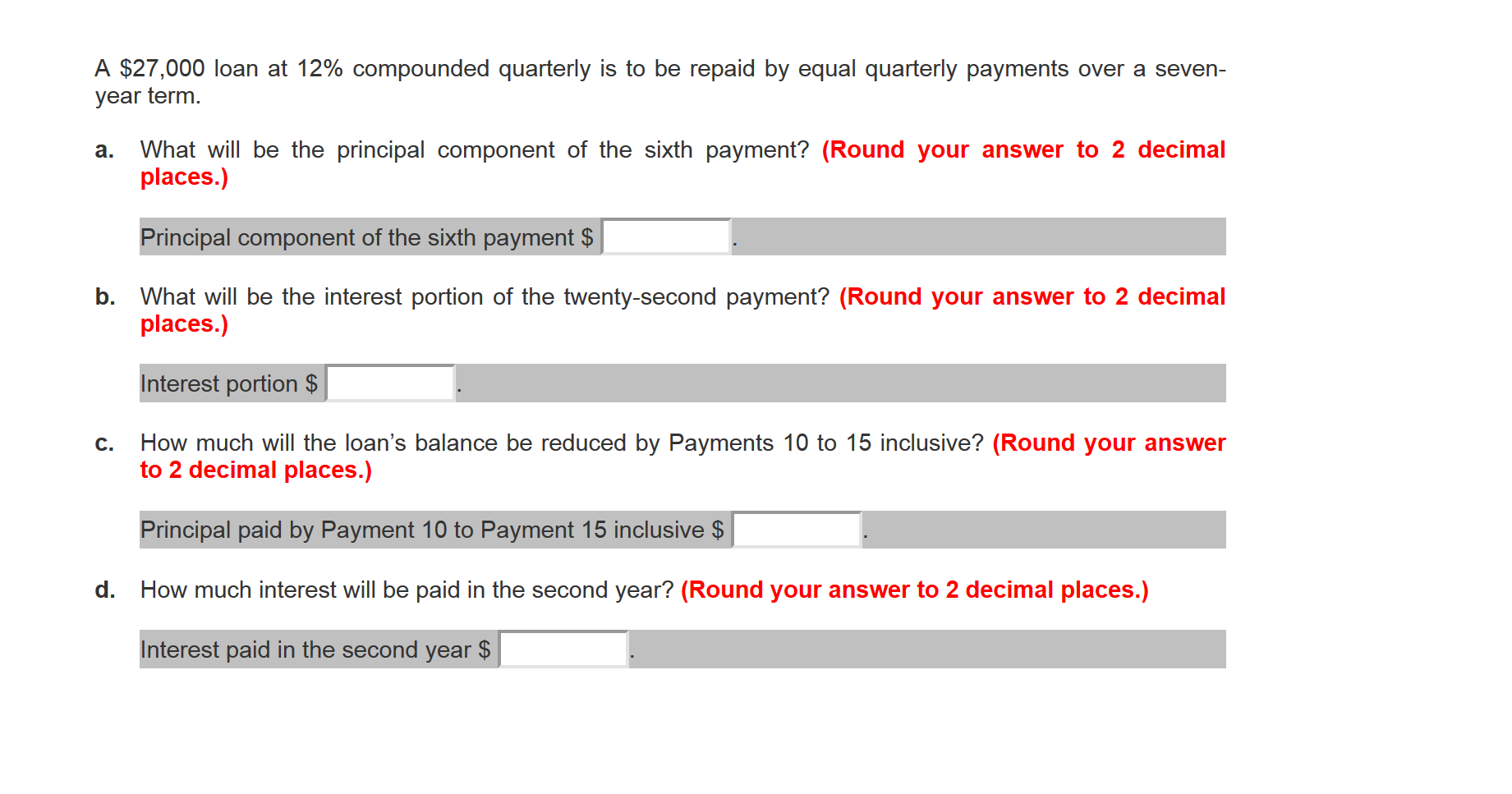A \$27,000 loan at 12% compounded quarterly is to be repaid by equal quarterly payments over a seven- year term. a. What will be the principal component of the sixth payment? (Round your answer to 2 decimal places.) Principal component of the sixth payment \$ b. What will be the interest portion of the twenty-second payment? (Round your answer to 2 decimal places.) Interest portion \$ C. How much will the loan's balance be reduced by Payments 10 to 15...

• ### A \$26,000 loan at 8% compounded quarterly is to be repaid by equal quarterly payments over...

A \$26,000 loan at 8% compounded quarterly is to be repaid by equal quarterly payments over a seven-year term. a. What will be the principal component of the sixth payment? (Round your answer to 2 decimal places.) Principal component of the sixth payment \$ . b. What will be the interest portion of the twenty-second payment? (Round your answer to 2 decimal places.) Interest portion \$ . c. How much will the loan’s balance be reduced by Payments 10 to...

• ### A \$27,000 loan at 8% compounded quarterly is to be repaid by equal quarterly payments over...

A \$27,000 loan at 8% compounded quarterly is to be repaid by equal quarterly payments over a seven-year term. a. What will be the principal component of the sixth payment? (Round your answer to 2 decimal places.) Principal component of the sixth payment \$ . b. What will be the interest portion of the twenty-second payment? (Round your answer to 2 decimal places.) Interest portion \$ . c. How much will the loan’s balance be reduced by Payments 10 to...

• ### A \$29,000 loan at 12% compounded quarterly is to be repaid by equal quarterly payments over...

A \$29,000 loan at 12% compounded quarterly is to be repaid by equal quarterly payments over a seven-year term. a. What will be the principal component of the sixth payment? (Round your answer to 2 decimal places.) Principal component of the sixth payment \$ . b. What will be the interest portion of the twenty-second payment? (Round your answer to 2 decimal places.) Interest portion \$ . c. How much will the loan’s balance be reduced by Payments 10 to...

• ### The interest rate on a \$100,000 loan is 7.5% compounded quarterly. What quarterly payments will reduce...

The interest rate on a \$100,000 loan is 7.5% compounded quarterly. What quarterly payments will reduce the balance to \$75,000 after five years? (Do not round intermediate calculations and round your final answer to 2 decimal places.) PMT \$

• ### A \$32,000 loan at 12% compounded quarterly is to be repaid by equal quarterly payments over...

A \$32,000 loan at 12% compounded quarterly is to be repaid by equal quarterly payments over a seven-year term. a. What will be the principal component of the sixth payment? (Round your answer to 2 decimal places.) b. What will be the interest portion of the twenty-second payment? (Round your answer to 2 decimal places.) c. How much will the loan’s balance be reduced by Payments 10 to 15 inclusive? (Round your answer to 2 decimal places.) d. How much...

• ### The interest rate for the first five years of an \$100,000 mortgage loan is 9.4% compounded...

The interest rate for the first five years of an \$100,000 mortgage loan is 9.4% compounded semiannually. Monthly payments are calculated using a 20-year amortization. a. What will be the principal balance at the end of the five-year term? (Do not round intermediate calculations and round your final answer to 2 decimal places.)   Principal balance \$      b. What will be the monthly payments if the loan is renewed at 6.8% compounded semiannually (and the original amortization period is continued)?...

• ### Janine borrowed \$35,000 from her bank. Monthly payments are \$600, including interest at 6.8% compounded quarterly....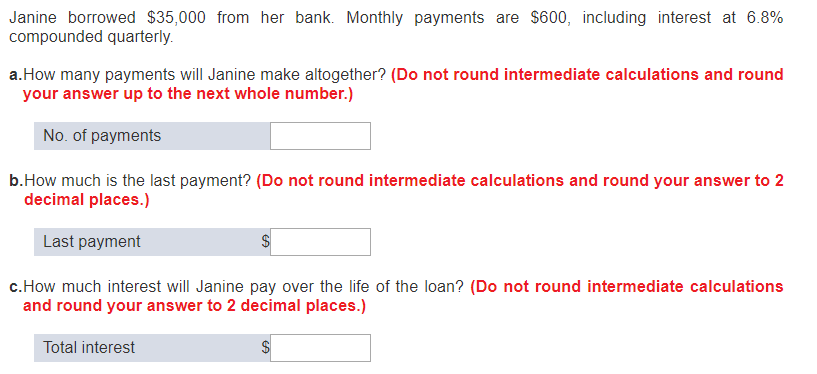Janine borrowed \$35,000 from her bank. Monthly payments are \$600, including interest at 6.8% compounded quarterly. a.How many payments will Janine make altogether? (Do not round intermediate calculations and round your answer up to the next whole number.) No. of payments b.How much is the last payment? (Do not round intermediate calculations and round your answer to 2 decimal places.) Last payment c.How much interest will Janine pay over the life of the loan? (Do not round intermediate calculations and...

• ### a loan \$1300 is being paid off by quarterly payments of \$110. if interest is at...

a loan \$1300 is being paid off by quarterly payments of \$110. if interest is at the rate of 6% compounded quarterly, how many full payment will be made?

Free Homework App